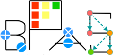Basic Polynomial Algebra Subprograms (BPAS)  v. 1.652
Factor< Ring > Class Template Reference

A Factor is a pair of a BPASRing element and an integer exponent. More...

`#include <Factors.hpp>`

Simplified semantic inheritance diagram for Factor< Ring >:Full inheritance diagram for Factor< Ring >:
[legend]

## Public Member Functions

Factor ()
Construct an empty factor.

Factor (const Ring &r, int e)
Construct a factor from a Ring element and an exponent. More...

Factor (Ring &&r, int e)
Move-construct a factor from a Ring element and an exponent. More...

Factor (const std::pair< Ring, int > &p)
Construct a Factor from a std::pair of a Ring element and integer exponent. More...

bool operator== (const Factor< Ring > &f)
Equality comparison operator. More...

bool operator!= (const Factor< Ring > &f)
Inequality comparison operator. More...

ExpressionTree convertToExpressionTree () const
Convert the Factor to an ExpressionTree. More...

## Friends

std::ostream & operator<< (std::ostream &out, const Factor< Ring > &f)
Output operator, defines a to string conversion. More...

## Detailed Description

### template<class Ring> class Factor< Ring >

A Factor is a pair of a BPASRing element and an integer exponent.

Template Ring should derive from BPASRing.

## ◆ Factor() [1/3]

template<class Ring>
 Factor< Ring >::Factor ( const Ring & r, int e )
inline

Construct a factor from a Ring element and an exponent.

Parameters
 r the Ring element e the exponent

## ◆ Factor() [2/3]

template<class Ring>
 Factor< Ring >::Factor ( Ring && r, int e )
inline

Move-construct a factor from a Ring element and an exponent.

Parameters
 r the Ring element e the exponent

## ◆ Factor() [3/3]

template<class Ring>
 Factor< Ring >::Factor ( const std::pair< Ring, int > & p )
inline

Construct a Factor from a std::pair of a Ring element and integer exponent.

Parameters
 p the pair

## ◆ convertToExpressionTree()

template<class Ring>
 ExpressionTree Factor< Ring >::convertToExpressionTree ( ) const
inlinevirtual

Convert the Factor to an ExpressionTree.

Returns
the ExpressionTree

Implements ExpressionTreeConvert.

## ◆ operator!=()

template<class Ring>
 bool Factor< Ring >::operator!= ( const Factor< Ring > & f )
inline

Inequality comparison operator.

Parameters
 f the Factor to compare
Returns
true iff *this != f.

## ◆ operator==()

template<class Ring>
 bool Factor< Ring >::operator== ( const Factor< Ring > & f )
inline

Equality comparison operator.

Parameters
 f the Factor to compare
Returns
true iff *this == f.

## ◆ operator<<

template<class Ring>
 std::ostream& operator<< ( std::ostream & out, const Factor< Ring > & f )
friend

Output operator, defines a to string conversion.

Parameters
 out the output stream f the Factor

The documentation for this class was generated from the following file: